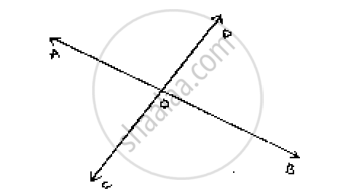# Two Lines Ab and Cd Intersect at O. If ∠Aoc + ∠Cob + ∠Bod = 270°, Find the Measures of ∠Aoc, ∠Cob, ∠Bod and ∠Doa. - Mathematics

Two lines AB and CD intersect at O. If ∠AOC + ∠COB + ∠BOD = 270°, find the
measures of ∠AOC, ∠COB, ∠BOD and ∠DOA.

#### SolutionGiven: ∠AOC + ∠COB + ∠BOP = 270°

To find: ∠AOC, ∠COB, ∠BOD and ∠DOA

Here, ∠AOC + ∠COB + ∠BOD + ∠AOD = 360°              [Complete angle]

⇒  270 + ∠AOD = 360°

⇒ ∠ AOD = 360° - 270°

⇒ ∠AOD = 90°

Now,

∠AOD + ∠BOD = 180°                [Linear pair]

90 + ∠BOD = 180°

⇒ ∠BOD = 180° - 90°

∴ ∠BOD = 90°

∠AOD = ∠BOC = 90°    [Vertically opposite angles]

∠BOD = ∠AOC = 90°  [Vertically opposite angles]

Concept: Concept of Parallel Lines
Is there an error in this question or solution?

#### APPEARS IN

RD Sharma Mathematics for Class 9
Chapter 10 Lines and Angles
Exercise 10.4 | Q 13 | Page 47